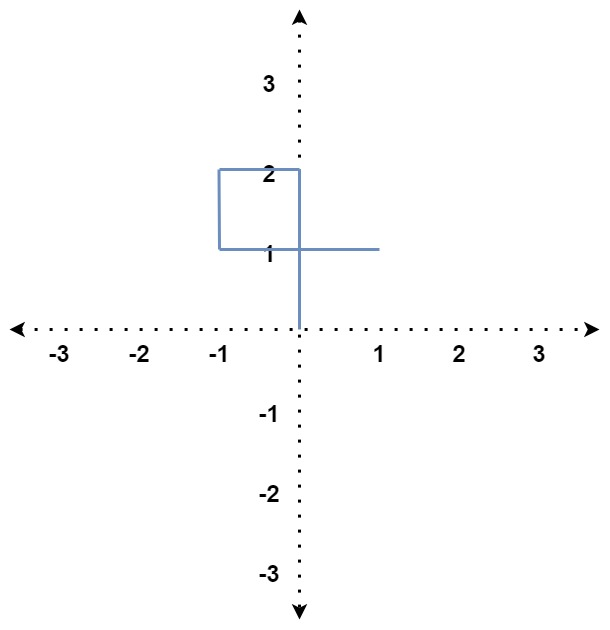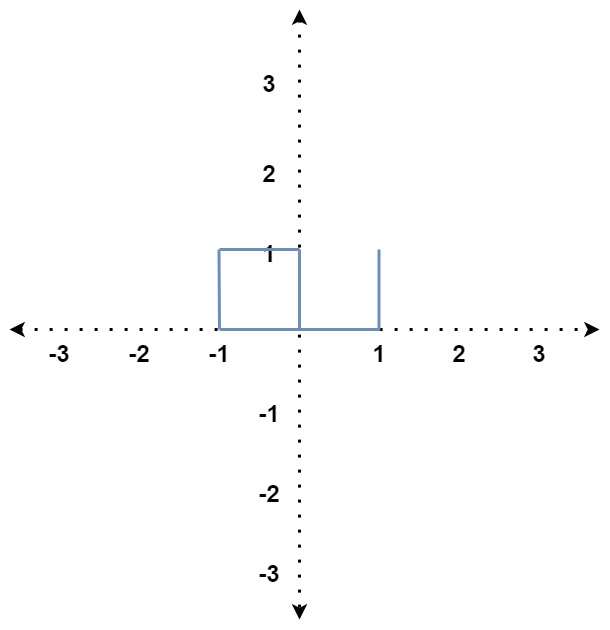335. Self Crossing
Hard
313
487

You are given an array of integers `distance`.

You start at the point `(0, 0)` on an X-Y plane, and you move `distance` meters to the north, then `distance` meters to the west, `distance` meters to the south, `distance` meters to the east, and so on. In other words, after each move, your direction changes counter-clockwise.

Return `true` if your path crosses itself or `false` if it does not.

Example 1:```Input: distance = [2,1,1,2]
Output: true
Explanation: The path crosses itself at the point (0, 1).
```

Example 2:```Input: distance = [1,2,3,4]
Output: false
Explanation: The path does not cross itself at any point.
```

Example 3:```Input: distance = [1,1,1,2,1]
Output: true
Explanation: The path crosses itself at the point (0, 0).
```

Constraints:

• `1 <= distance.length <= 105`
• `1 <= distance[i] <= 105`
Accepted
30.1K
Submissions
102.3K
Acceptance Rate
29.5%

Seen this question in a real interview before?
1/4
Yes
No

Discussion (0)

Related Topics